Courses

# Structural Analysis And Steel Structures - 2

## 20 Questions MCQ Test Mock Test Series for Civil Engineering (CE) GATE 2020 | Structural Analysis And Steel Structures - 2

Description
This mock test of Structural Analysis And Steel Structures - 2 for GATE helps you for every GATE entrance exam. This contains 20 Multiple Choice Questions for GATE Structural Analysis And Steel Structures - 2 (mcq) to study with solutions a complete question bank. The solved questions answers in this Structural Analysis And Steel Structures - 2 quiz give you a good mix of easy questions and tough questions. GATE students definitely take this Structural Analysis And Steel Structures - 2 exercise for a better result in the exam. You can find other Structural Analysis And Steel Structures - 2 extra questions, long questions & short questions for GATE on EduRev as well by searching above.
QUESTION: 1

Solution:
QUESTION: 2

### When the depth of a simply supported beam carrying a concentrated load at the midspan is doubled, the deflection of the beam at midspan will change by a change of

Solution: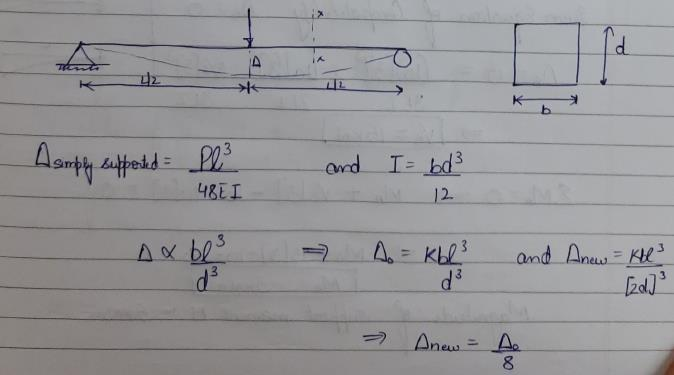QUESTION: 3

### An applied couple M is moving on a simply supported beam of span L as shown below. Hence the absolute bending moment developed in the beam will be.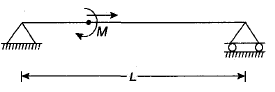Solution:
*Answer can only contain numeric values
QUESTION: 4

Rivets and bolts subjected to both shear stress ( τvf ,cal ) and axial tensile stress ( σσf ,cal ) shall be so proportioned that the stresses do not exceed the respective allowable stresses τvf and σtf , and the value of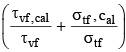does not exceed __________

Solution:
*Answer can only contain numeric values
QUESTION: 5

The maximum slenderness ratio of compression members resulting from wind or seismic force only, provided deformation of such members does not adversely affect the stress in any part of the structure is __________

Solution:
QUESTION: 6

As the slenderness ratio of column increases, the value of allowable stress

Solution:
QUESTION: 7

A joint in the length of a column is known as

Solution:
QUESTION: 8

In rolled steel beam section, the shear force is resisted by

Solution:
QUESTION: 9

Bearing stiffeners are provided to avoid

Solution:
QUESTION: 10

The effective length of a fillet weld of length L and size s is given by

Solution:
QUESTION: 11

In the portal frame shown below, what are the distribution factors for members BA, BC and BD respectively?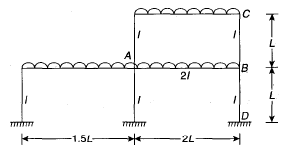Solution: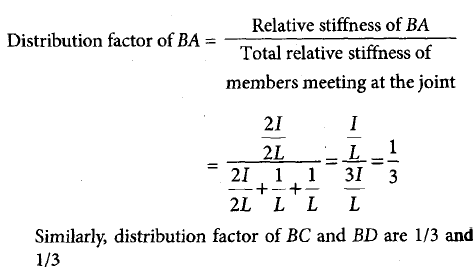QUESTION: 12

For the rigid frame shown below, what is the moment reaction at A?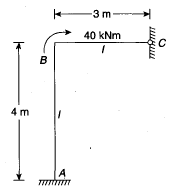Solution: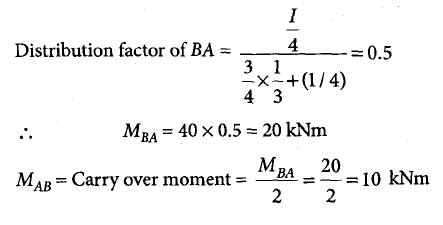QUESTION: 13

For the fixed beam shown below, what is the fixed end moment at A for the given loading?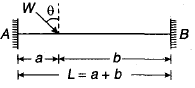Solution: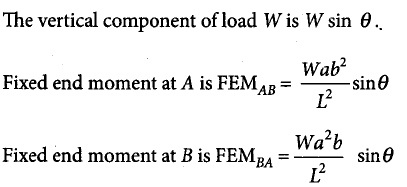QUESTION: 14

The effective length of column AB shown below is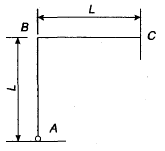Solution:
QUESTION: 15

Four bolts share the load P as shown in the given figure. The shear strength of bolts is 30 kN and tension strength of bolt is 40 kN. Which one of the following is the value of P?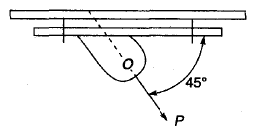Solution: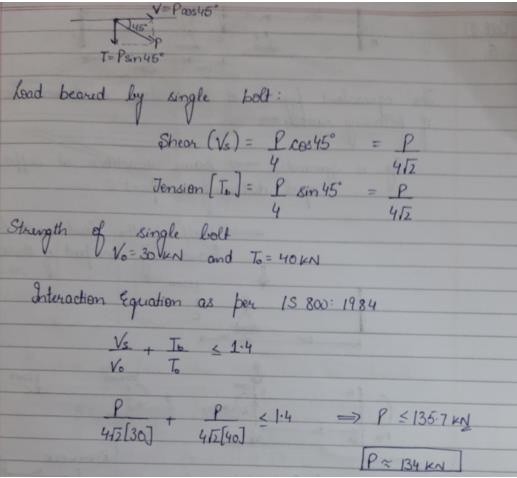*Answer can only contain numeric values
QUESTION: 16

The allowable tensile stress is 150 N/mm2; the capacity of single ISA 100 × 100 × 10 mm as tension member connected by one leg using 6 number of 21.5 mm gross rivets is _________ kN.

Solution: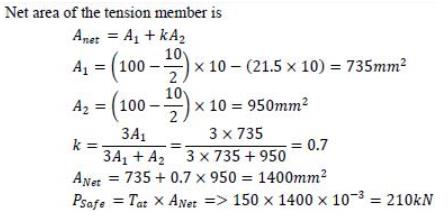*Answer can only contain numeric values
QUESTION: 17

The 6 mm fillet welds used for a joint is shown in the given figure. The permissible shear stress in the weld is 110 N/mm2. Then, the minimum lap length required will be __________ mm.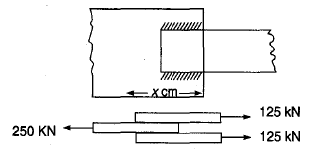Solution: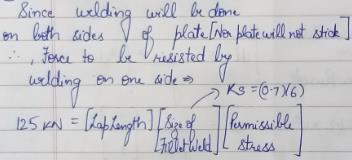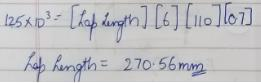QUESTION: 18

A single riveted lap joint fails due to which of the following? 1. Tearing 2. Bearing 3. Shearing

Solution:
QUESTION: 19

Consider the following assumptions:

1. The shearing stress in all the rivets is uniform

2. The bearing stress is not uniform

3. Bending of rivets can be neglected

The assumptions made while designing a riveted strength would include

Solution:
QUESTION: 20

The following observation related to designing of laced column:

1. Single lacing systems on opposite planes shall not exceed 180

2. Lacing bar should be a flat

3. The slenderness ratio of the lacing bar for compression shall not exceed 180

4. Laced compression member are not provided with tie plates of end

Of these observations:

Solution: